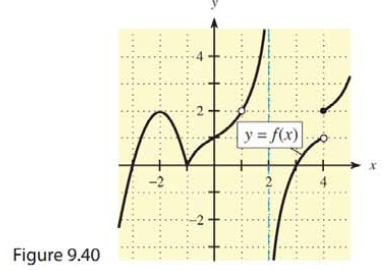Chapter 9, Problem 44REMathematical Applications for the ...

12th Edition
Ronald J. Harshbarger + 1 other
ISBN: 9781337625340

Solutions

Chapter
SectionMathematical Applications for the ...

12th Edition
Ronald J. Harshbarger + 1 other
ISBN: 9781337625340
Textbook Problem

Use the graph of y = f(x) in Figure 9.40 on the previous page to answer the questions in Problems 44-46.Explain which is greater: the average rate of change of f over [ -3, 0 ]  or over  [ -1, 0 ] .

To determine

The greater average rate change of function, f from f over [3,0] or f over [1,0].

Explanation

Given Information:

The provided graph of the function is,

Explanation:

Consider the provided graph of function,

For f over [3,0], apply the formula for average rate of change,

Avg=f(0)f(3)0(3)

From the graph, substitute 1 for f(0) and 0 for f(3),

Avg=100(3)=13

For

Still sussing out bartleby?

Check out a sample textbook solution.

See a sample solution

The Solution to Your Study Problems

Bartleby provides explanations to thousands of textbook problems written by our experts, many with advanced degrees!

Get Started

Calculate y'. 45. y = ln( cosh 3x)

Single Variable Calculus: Early Transcendentals, Volume I

solve the equation by using the quadratic formula. 138. x2 6x + 6 = 0

Applied Calculus for the Managerial, Life, and Social Sciences: A Brief Approach

Find for x = 3t2 + 1, y = t6 + 6t5. t4 + 5t3 4t3 + 15t2

Study Guide for Stewart's Multivariable Calculus, 8th